# Make Math Matter With Concreteness Fading

## Importance of Context and Concrete Manipulatives From Kindergarten Through Grade 12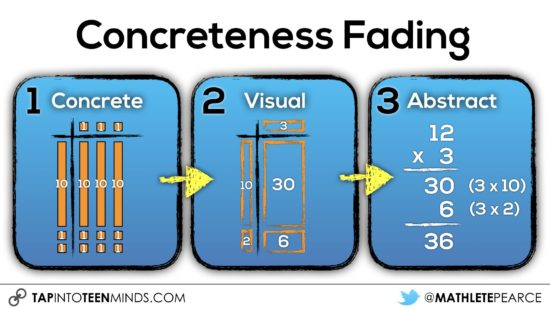During the first half of my teaching career, I would spend what seemed to be the first half of a math lesson teaching a new math concept by sharing definitions, formulas, steps and procedures. To make things more challenging for my students, I would simultaneously introduce the symbolic notation used to represent those ideas. Then, I would spend the remainder of the lesson attempting to help my students make sense of these very new and often abstract ideas. By the end of the lesson, I could help many students build an understanding, but there was always a group I felt who I would leave behind.

Like many other teachers, I was just teaching in a very similar way to that how I was taught.

I knew no different.

However, if we consider that new learning requires the linking of new information with information they already know and understand, we should be intentionally planning our lessons with this in mind. A great place to start new learning is through the use of a meaningful context and utilizing concrete manipulatives that students can touch and feel. When we teach in this way, we minimize the level of abstraction so students can focus their working memory on the new idea being introduced in a meaningful way.

## Concreteness Fading: Concrete, Representational, Abstract (CRA) Approach

When we intentionally start with concrete manipulatives to learn new math concepts, our goal is to help students better construct an understanding of the mathematics in their mind. The goal is not to burden students with a big bag of manipulatives that they must carry around with them anytime they are required to do any mathematical thinking, but rather to ensure that they can build their spatial reasoning skills physically – through the manipulation of concrete objects – so they can begin to visualize mathematics in their mind. When a student is able to “look up” as if they are peering into their mind to visualize their math thinking, we know students are thinking conceptually rather than simply following a memorized procedure.

While students are working with concrete manipulatives, it is helpful for the teacher to model visual representations of the student work for all to see. By introducing these visual drawings of the concrete representations students are creating, it will be easier for students to shift away from concrete manipulatives and towards visual (drawn) representations when they are ready.

When students have built an understanding both concretely and visually, we can then begin moving to the final stage called abstraction where we use symbolic notation. The goal here is that when students use the symbolic notation, they can visualize what the concrete representation of that mathematical statement represents.

Some know this idea as concreteness fading, while others have called this progression concrete, representational, abstract (CRA). In either case, the big idea is the same. Start with concrete manipulatives, progress to drawing those representations and finally, represent the mathematical thinking abstractly through symbolic notation.

Let’s look at a couple different questions at different grade levels where using context and concrete manipulatives can lower the floor and help us progress towards more abstract representations.

## Scenario #1: Elementary / Primary Classroom

If we were to ask students in a grade 2 class, I might have them look at the following image and ask them what they notice and what they wonder: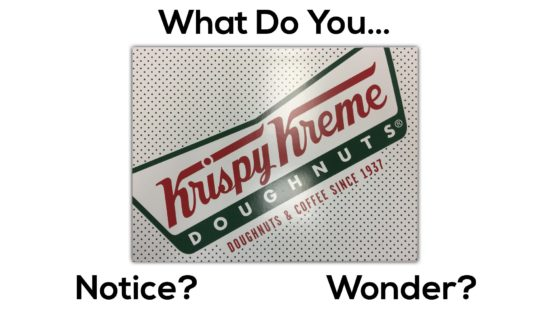Then, I might have them predict how many doughnuts they believe fit in that box.

After students talk with a neighbour and share out their predictions, I would say:

This box of doughnuts has 3 rows of 4 doughnuts.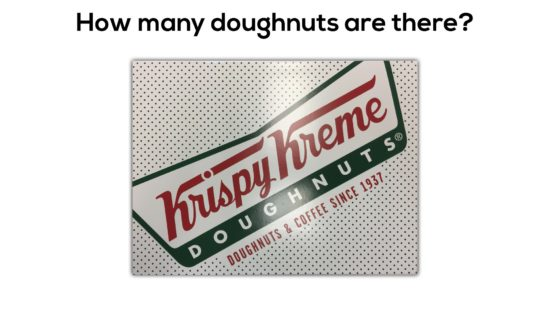This question may seem quite simplistic after giving this new information, however for students who are just beginning to shape their understanding of number including place value and additive thinking, we are now throwing a very heavy and abstract idea at them.

In a perfect world, we could give them real doughnuts (or bagels, to be health conscious) so they could recreate the situation right in front of them: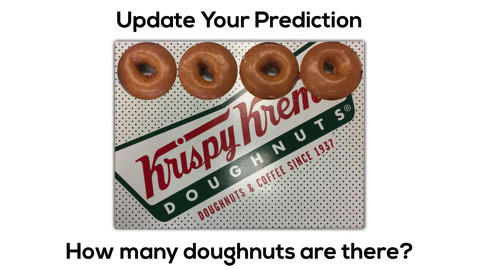Despite the fact that using square tiles or circular counters to represent doughnuts is more concrete than drawing doughnuts or using symbols (numbers and operations), we must understand that concrete manipulatives are still more abstract than using the actual items in the quantity being measured.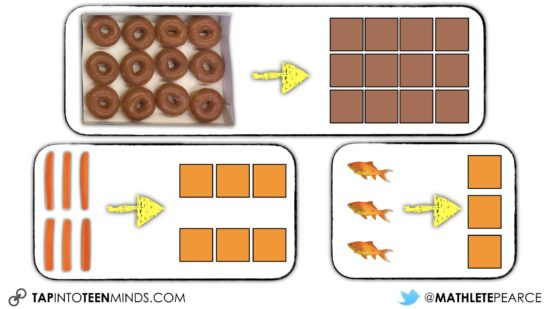As students understanding of number increases, so too should their ability to begin using concrete manipulatives instead of real doughnuts to work through this situation.

As students use concrete manipulatives to build their conceptual understanding of a new idea, they will begin to feel burdened by the manipulatives and seek out less cumbersome tools and representations to show their thinking. If the teacher has been drawing visual representations of the concrete representations students share with the group along the way, many will eventually transition to drawing their representations rather than building them concretely. However, for other students who have seemingly mastered the concrete representations but are not shifting to visuals, we may need to help scaffold them along.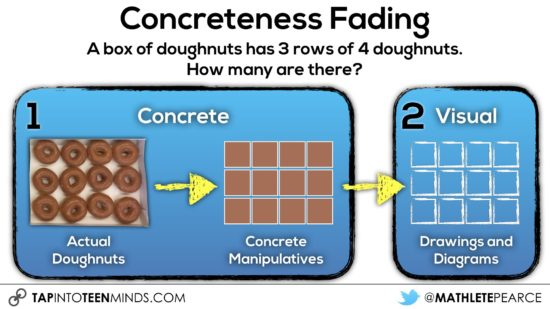With conceptual understanding continuing to deepen through the use of drawn visual representations, teachers can continue sharing student thinking through the use of visuals and begin introducing symbolic notation. Since students have had a significant amount of time to inquire, investigate and solve problems using both concrete and visual representations, they will develop the ability to visualize representations in their mind. At this stage, it would seem more efficient to use symbolic notation such as numbers and operations to represent mathematical thinking rather than building concretely or drawing visually.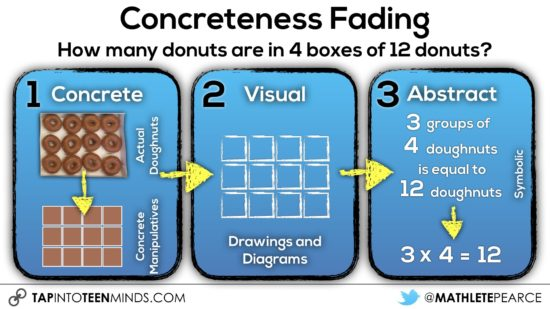It is important to note that while the concreteness fading model or concrete, representational, abstract (CRA) approach is a general progression that we want to keep in mind when teaching new concepts in math class, we don’t want to overthink it either.

For example, in the abstract / symbolic phase, you’ll notice the words:

“3 groups of 4 doughnuts is equal to 12 doughnuts”

By no means am I suggesting that we should wait until the concrete and visual phases are mastered before using those words. I would actually suggest that we are verbally saying those words during the concrete stage and even possibly writing down that sentence during the concrete stage since there are no new symbols or abstract ideas being introduced by doing so. With an idea like single digit multiplication, you might consider having students build the concrete representations and the teacher may draw the visual representation as well as the symbolic representations at the same time.

The key with concreteness fading is that we are aware of these three phases and we use our professional judgement to determine when to introduce each phase as to push student thinking forward without overwhelming them with too much abstraction too quickly.

## Scenario #2: Upper Elementary Classroom

We could introduce a similar question for say a grade 4 class by simply increasing the complexity of the question such as:

How many doughnuts are in 3 boxes?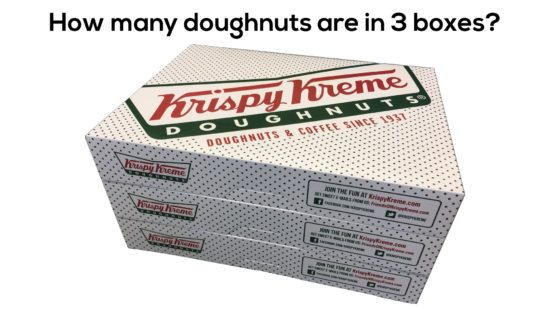If we are asking students to work with a problem that we could consider is a multi-step multiplication problem, the beginnings of volume or a double digit by single digit multiplication problem, my hope would be that students are now comfortable abstractly using concrete manipulatives (connecting cubes, square tiles, etc.) to represent how many doughnuts are in 3 boxes. If a student is struggling with the abstraction of using a concrete manipulative in place of the actual object – like doughnuts in this case – we might need to reassess the readiness of this particular student and do some more work with more accessible problems.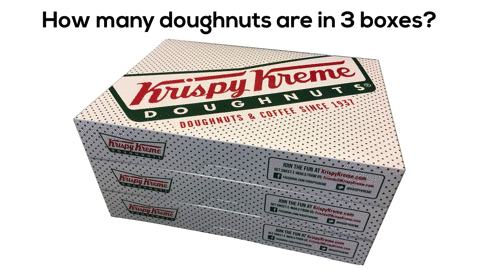In this particular case, the progression of concreteness fading might look something like the following: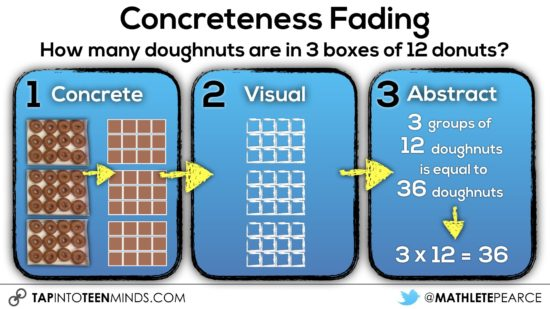Or students might go about it using their knowledge of arrays and extend the idea to area models before finally developing a student generated algorithm: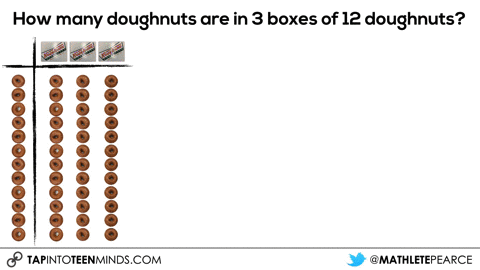Here’s a summary of the concreteness fading progression that may take place if students have been doing work with arrays and area models: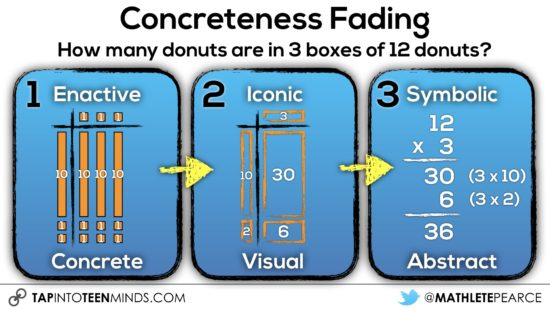Assuming students have had a substantial amount of experience building concrete representations of multiplication, you may see students skipping right over the concrete phase to the visual stage creating drawn diagrams of this situation. This is absolutely fine as a student who is able to draw what the concrete representation should look like suggests that she could indeed build that representation if required. Furthermore, this also suggests that this student is now able to create a more abstract representation of that concrete model, which is what we are hoping to develop.

What I would not advocate is completely skipping over the first two phases and focusing only on the symbolic representation. Despite the fact that some students may have a visual of that concrete model clear in their minds, we don’t want to promote students relying solely on procedural fluency and risk forgetting all of that conceptual understanding we worked so hard to build. By giving students enough practice drawing visual as well as abstract or symbolic representations, they are utilizing their conceptual understanding and procedural fluency in tandem, where they can be used most effectively.

As students become more comfortable with the abstract representation of multiplying 2-digit numbers by 1-digit numbers, we might think it is fine to start with the abstract stage of concreteness fading when we progress to 2-digit by 2-digit multiplication. Although we have progressed through the stages of concreteness fading for one concept, as we add a new level of complexity (i.e.: adding another digit to multiplication) we should be cycling back to the concrete stage to lower the floor for all students to access this new learning.

A great example that works well here is the 3 act math task, Doughnut Delight.

After students notice and wonder, we land on the question:

How many doughnuts are in that giant box?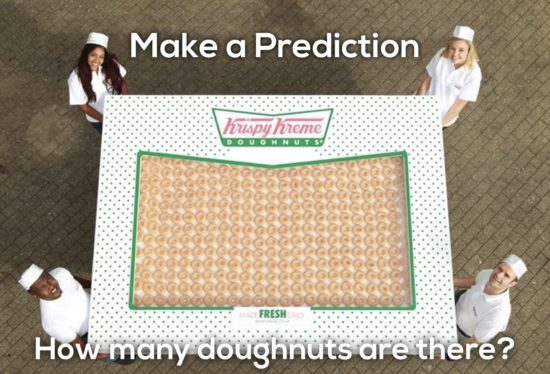After students make predictions and justify their reasoning, I reveal the dimensions to the students:

There are 32 rows and 25 columns of doughnuts.

They are then set off to find a solution using an effective strategy of their choosing. Assuming this is their first exposure to 2-digit by 2-digit multiplication, starting in the concrete stage using base-10 blocks would be appropriate. For students who may already have made connections to the work they have done previously, they may choose to draw out an array or use another visual model to show their thinking.

Here in Ontario, we explicitly introduce 2-digit by 2-digit multiplication in grade 5. Every time I’ve used this task with students in grade 5, most are rushing to the algorithm and making errors due to the lack of conceptual understanding.

I do my best to try and get students to back up a stage or two in order to truly understand the mathematics we are asking them to grapple with. This can be a struggle, because often times students just want to get “an answer” and move on.

However, if they are shown how easy multiplication can be by having a conceptual understanding in their back pocket, they will eventually jump on the opportunity.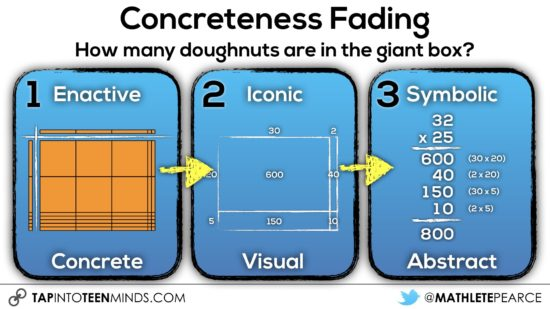## Scenario #3: Middle School Classroom

In a middle school classroom (end of junior/intermediate classroom in Ontario), the question might sound more like this:

There are 36 doughnuts in 3 boxes.
How many doughnuts are in 7 boxes?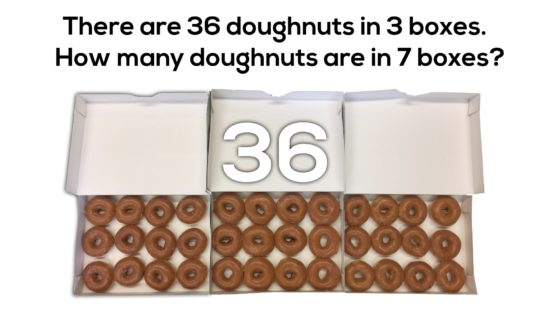While this may seem like a lot of doughnuts for students to represent concretely, having linking cubes, square tiles or other tools students can use to organize their thinking is important especially for those who have not yet built a conceptual understanding of what this task is asking of them. In this case, we are exploring a proportional relationship where the number of doughnuts is proportional to the count of how many boxes there are.

Despite the fact that proportional reasoning is introduced explicitly in the Ontario Grade 4 Math Curriculum, many of our grade 9 students continue to struggle with this type of reasoning. Many may not have fully conceptualized the prior knowledge necessary for them to be successful at that particular grade level. Before I understood the power of concrete and visual representations, I can recall trying to help students in my grade 9 (and sometimes grade 10) class by breaking down the symbolic representation with more symbols.

For example, with this particular problem, I might have attempted unpacking the problem with students by creating a proportion and solving for the unknown: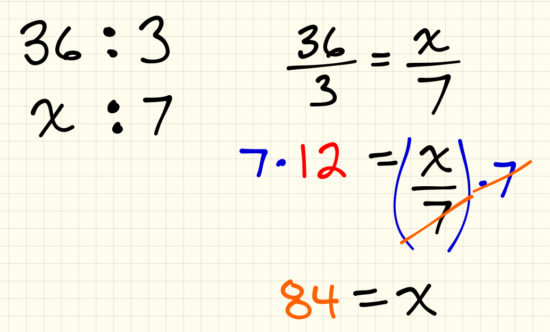While working with and solving for unknowns in a proportional relationship was an expectation in my curriculum and in the curriculum prior to grade 9, I was stuck in my habit of trying to start with abstract symbols and unpacking them. However, the reality is that when we do this sort of work without building the necessary conceptual understanding at the concrete and visual phases of concreteness fading, students are forced to either memorize the steps and procedures or get left behind. For some students, they are able to make their own connections at the symbolic stage based on their prior knowledge from past experiences in school and at home, while other students are left scrambling to understand with stress and anxiety levels building with each passing class.

How might I have approached this same problem had I known and understood the concreteness fading model?

Well, I would definitely start with concrete manipulatives for all of my students. Just because a student is able to solve familiar problems using all the right steps and procedures does not necessarily mean that they have a conceptual understanding of the mathematics they are employing.

One possible idea could be giving students connecting cubes and having them model out the situation. They might start by grabbing 36 cubes and dividing them to the 3 boxes. Then, they could double the 3 boxes of doughnuts to get 6 boxes and add an additional box.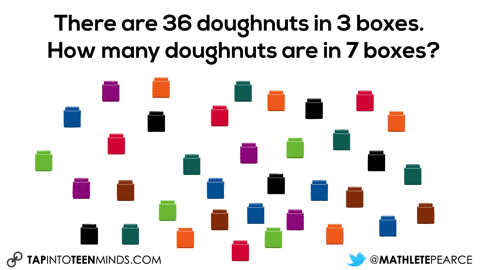Some professional noticing you will want to engage in would be determining whether students are using additive thinking, multiplicative thinking or a combination of the two. To build an understanding of proportional reasoning, we must help students to think multiplicatively. So while thinking additively is not bad or wrong, we do want to try to prompt students to think multiplicatively.

For example, you might ask students:

How many times bigger is the quantity in 7 boxes than in 1 box (i.e.: 7 times bigger)?

How many times bigger is the quantity in 7 boxes than the quantity in 3 boxes? (i.e.: 2 and 1 one-third times bigger)

While I try to encourage all students to make a concrete model, some may be moving away from physical manipulatives and pushing towards a visual model which would suggest that they are ready to move one step deeper into abstraction.

Here’s an example of how a double number line could be used to help students visualize the situation and problem solve their way to a solution.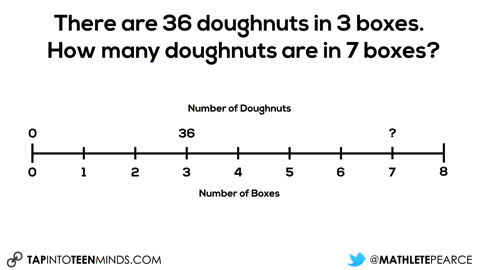From both the concrete models and the visual models students use in the classroom, I can prompt students to attempt modelling their thinking using symbolic notation such as algebraic expressions and equations.

The more I can help my students link their concrete and visual models to more abstract representations, the stronger their conceptual understanding will be to help support any procedural approaches they wish to use to progress towards more efficient methods.

Had I known more about concreteness fading earlier in my career, the progression might have looked more like this: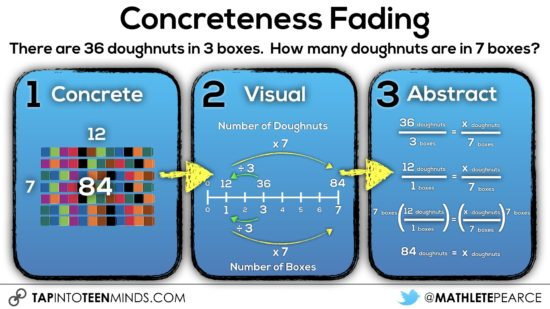While the above concreteness fading progression would have been a huge help to all students in my class to better understand proportional relationships, I would later learn from my colleagues in the AMP group that setting up a proportion of equivalent fractions is not a very powerful method mathematically, since it yields only the numerical answer to a single problem. A more powerful approach is to uncover the proportional relationship in the problem situation, since this allows us to immediately solve any problem based on that situation.

Let’s take a closer look.

## Fuel Sense Making: Scaling in Tandem and The Constant of Proportionality

I have been blessed to be a part of an amazing group of mathematicians funded through the Arizona Mathematics Project (AMP) to make sense of proportional relationships and this group of 18 mathematics education influencers have landed on some really useful definitions related to this very commonly encountered type of middle school math problem.

When we look at the animation of the concrete model using connecting cubes, you can see the two methods of attacking proportional relationships that the AMP group refers to as:

• scaling in tandem; and,
• using the constant of proportionality.

We can see the use of scaling in tandem when we see the doubling the number of boxes and number of doughnuts from 3 boxes, 36 doughnuts to 6 boxes, 72 doughnuts.

We can see the use of the constant of proportionality when we look at the number of doughnuts (12) in a single box – often referred to as the unit rate.

First, we will head a bit further down the concreteness fading continuum by taking our horizontal double number line and represent it as a vertical number line. This is a nice way to progress towards a table of values without losing the relative magnitude between each quantity on the number line.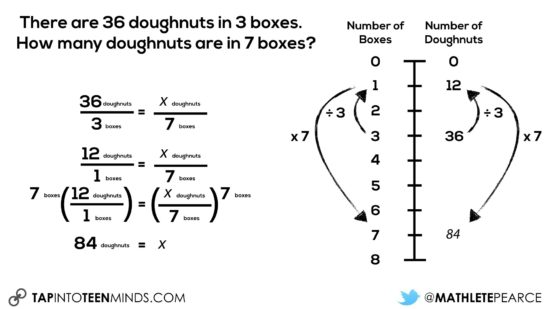For years, I would teach my students with an end goal of setting up and solving a proportion rather than focusing on helping them “own the problem” as Dick Stanley put it at our recent AMP meeting.

What we are referring to here is the limited usefulness of setting up a single proportion for a “rule of 3” problem.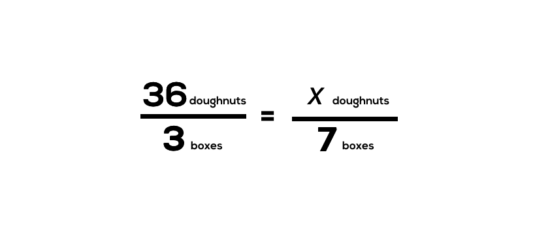When we set up a proportion of equivalent fractions, we have set out to solve a single problem and often times, we unintentionally rush to a procedure by setting up and solving for a single unknown. While this might be efficient for finding a single answer to a closed problem, it does not help us efficiently solve multiple problems nor does it promote a deep conceptual understanding of the proportional relationship that underpins this situation.

While I do not want to advocate that we avoid proportions altogether, I would much prefer giving students the opportunity to explore these problems more deeply and allow for the students to stumble upon some of the procedures we see taught explicitly in middle school classrooms.

Let’s look at where we might start.

## Ratio Reasoning: Scaling in Tandem

We can see from the animation below that scaling in tandem is responsible for allowing us to solve a proportion using any of the procedures we see taught in many middle school math classrooms: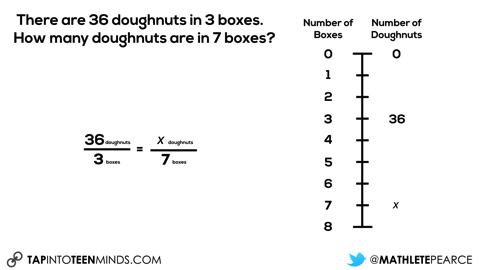By utilizing ratio reasoning by scaling in tandem, students are explicitly introduced to the power of the proportion, but in a much more powerful way.

If students are encouraged to utilize scaling in tandem with double number lines, tables and equivalent fractions, over time we can help students see that some of this scaling can be done more efficiently: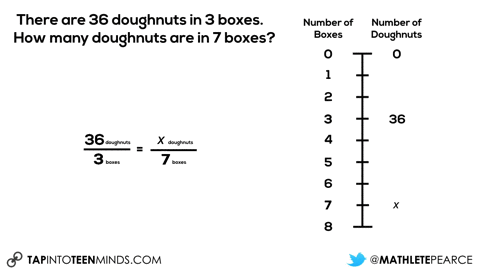Over time, students may progress from a vertical number line to a table of values. When students are ready, they may begin disregarding the magnitude of number allowing them to “skip over” some of the values on the number line.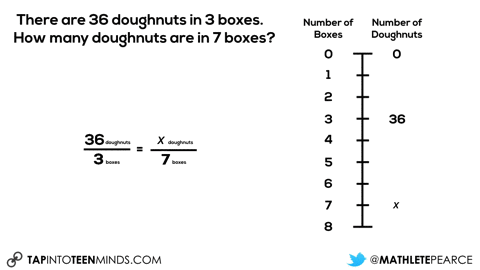We can use this scaling in tandem strategy to find any unknown in this proportional relationship, but it will take a bit of work.

For example, we can find the number of doughnuts in 9 boxes of doughnuts by scaling in tandem by multiplying both 36 doughnuts and 3 boxes by 9/3 or 3: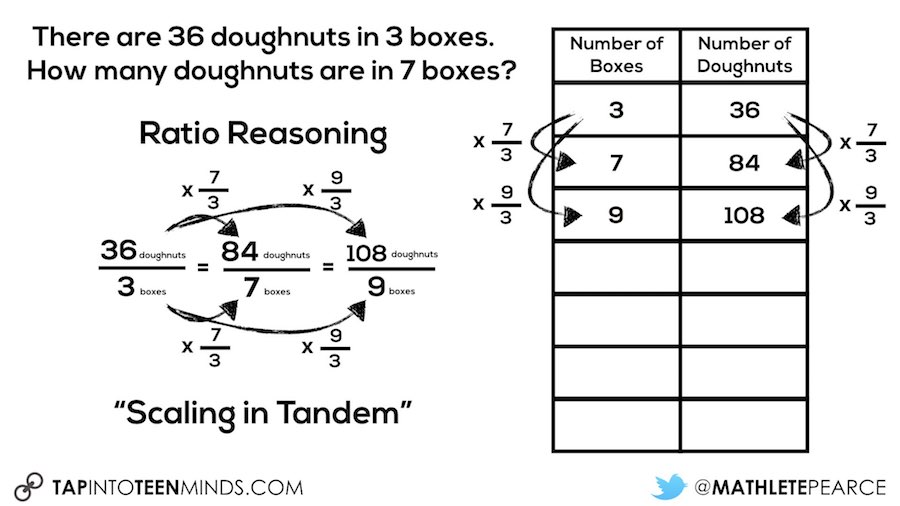As students become more fluent using scaling in tandem as a strategy for proportional relationships, we can then begin making generalizations: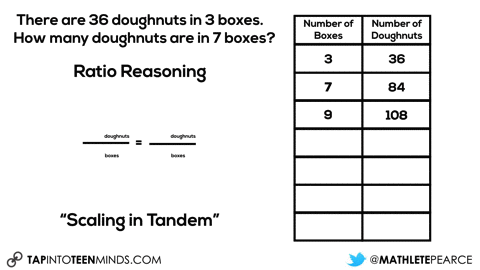What we see through this generalization is the conceptual understanding for why the common procedures we see in math classrooms actually work.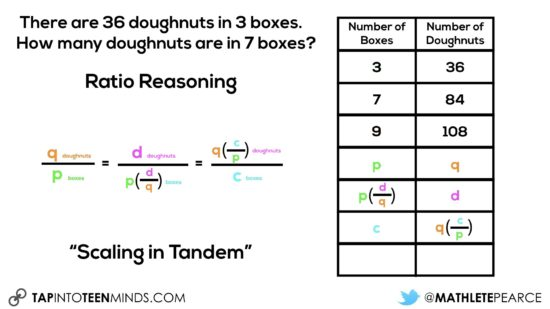One of the most over-used, but misunderstood tricks from the middle school math classroom is cross multiplication. When we look at the generalization of scaling in tandem, we can see where ideas like cross multiplication comes from:

q/p = d/c

qc = pd

c = pd/q

or

d = qc/p

While I taught tricks like cross-multiplication, “the magic circle” and “y-thingy-thingy” before I constructed a firm conceptual understanding of proportional relationships, the reality is that they provide a dead end pathway to a single answer rather than an understanding that allows you to own the problem.

So rather than simply teaching a trick using steps and procedures, let’s give students an opportunity to build a conceptual understanding of scaling in tandem and challenge them to come up with their own procedures and algorithms.

## Rate Reasoning Using the Constant of Proportionality

While we can use ratio reasoning and scaling in tandem to up the conceptual understanding over solving a “rule of 3” problem using a proportion, we still don’t “own” the problem yet.

Under the hood of every proportional relationship lies a constant that we can use to solve ANY problem related to the proportional situation. This constant of proportionality can be found by taking the quotient of any two covarying values in the relationship.

Many know this constant of proportionality as the unit rate.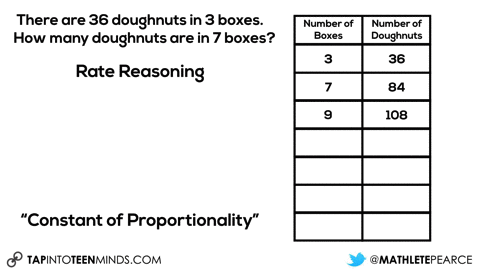Unlike the ratio reasoning strategy of scaling in tandem where one must determine a new scale factor to find each unknown quantity in a proportional relationship, the rate reasoning strategy of finding the constant of proportionality allows one to use the constant to find any unknown from the relationship.

In the case demonstrated here where the number of doughnuts is proportional to the number of boxes, we can determine the number of doughnuts in any number of boxes by multiplying the number of boxes by 12 doughnuts per box, while we can determine any number of boxes by multiplying the number of doughnuts by 1/12 boxes per doughnut (or dividing by 12 doughnuts per box).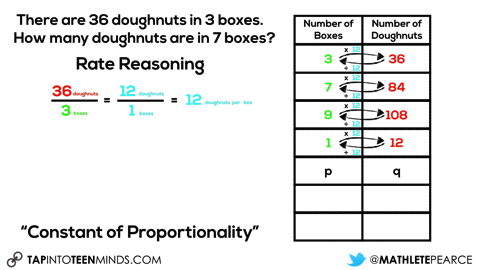So again, while I see huge value in students understanding how they can use ratio reasoning to scale in tandem to solve problems involving proportional relationships, only when we unlock the conceptual understanding behind rate reasoning and the constant of proportionality do we own every problem related to that proportional relationship.So rather than suggesting that the concreteness fading progression should end at the creation of a proportion of equivalent fractions and solving for an unknown, I would much rather see students exploring both ratio reasoning by scaling in tandem and rate reasoning through the constant of proportionality. Therefore, a suitable progression might look something like this: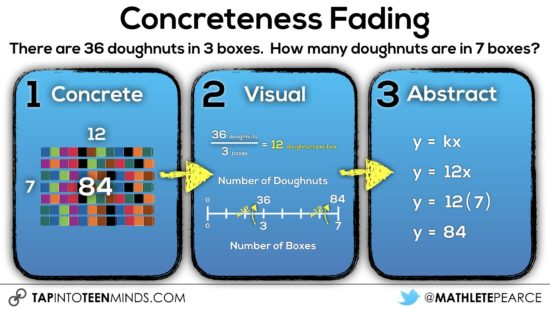## Scenario #4: Middle and High School Classroom

In grade 8 classrooms here in Ontario, we start making a serious push towards deeper algebraic reasoning and functional thinking which carries over into the deep exploration of linear and quadratic relationships in grades 9 and 10. While the context for doughnuts may not be my favourite – despite all their yummy-ness – it is possible for us to extend our thinking around this context from multiplicative and proportional reasoning to algebraic and functional thinking.

In this case, we’re going to continue exploring some situations where the goal is to figure out:

How many doughnuts are there?

In the first scenario, we’re looking at a 1 row box or “strip” of doughnuts, however we do not know how many are in each box initially: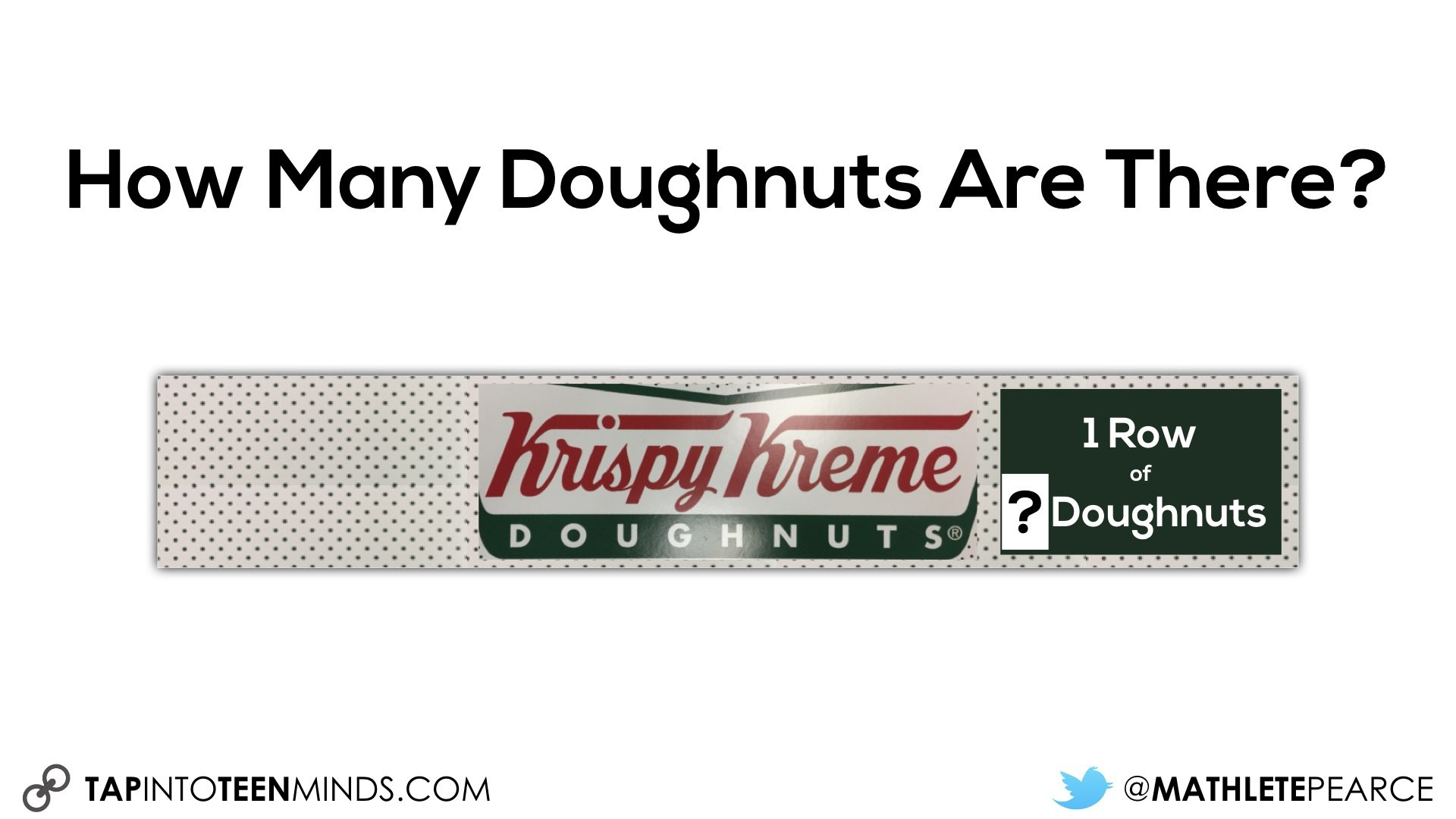We are then given an opportunity to take a guess at how many might be in that box considering the width of the box should be approximately the width of 1 doughnut.

Since a proportional relationship exists between the number of doughnuts and number of boxes, we can use our understanding of the constant of proportionality to help us create an equation for this situation.

If we assume (or we are given) the number of doughnuts in each box, we can then determine how many doughnuts in total.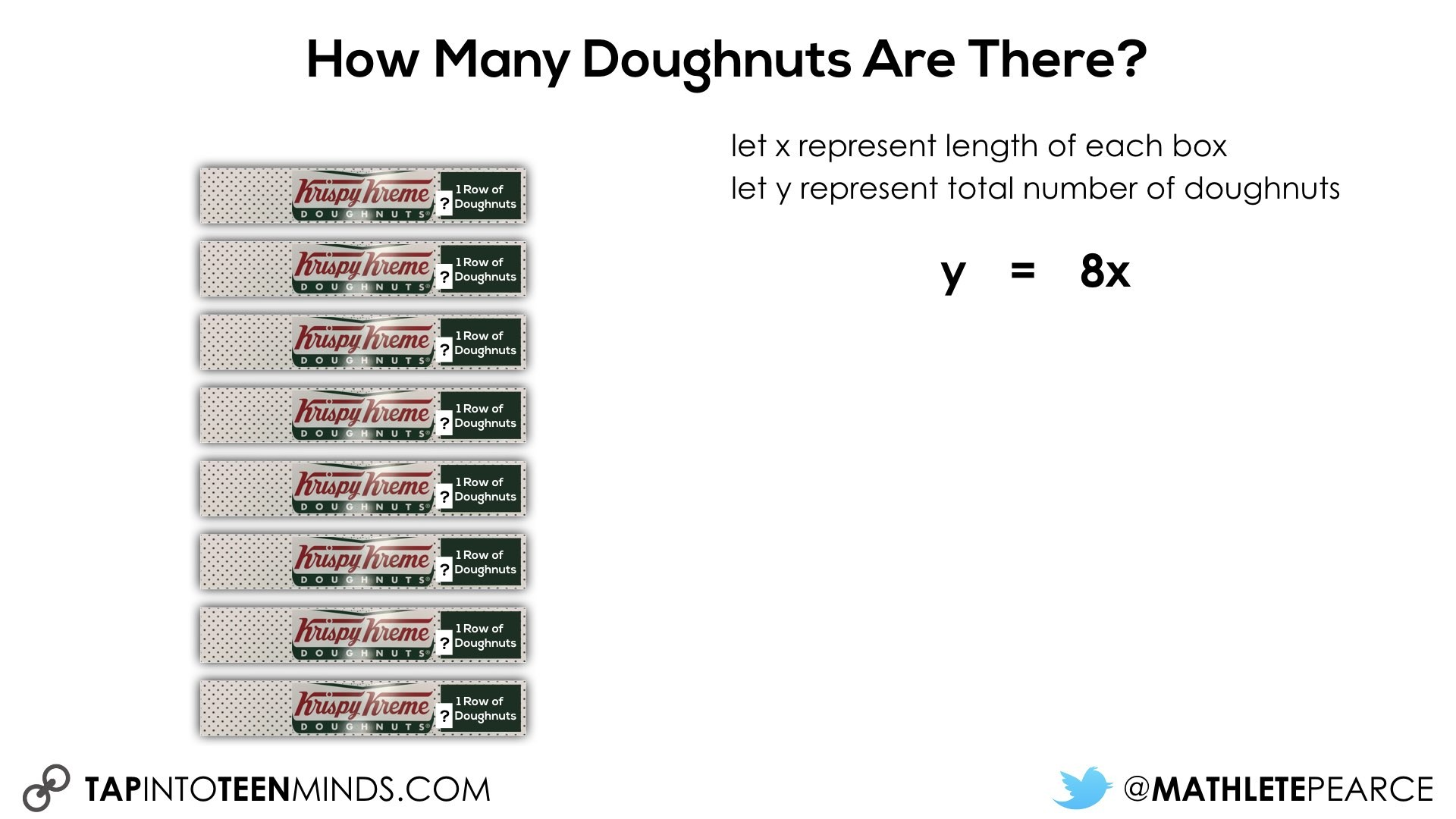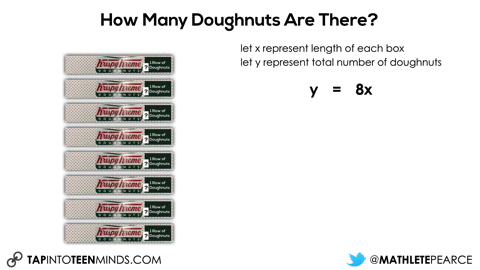We can also use this same proportional relationship with the same situation where the total number of doughnuts in 8 longer boxes is known in order to determine the number of doughnuts in each box: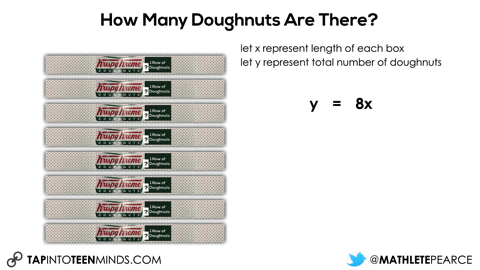Not super interesting, I know. However, I’d like to extend this thinking further to help us dive deeper into algebraic thinking with concrete and visual representations in mind.

In this next situation, we are given 8 square boxes and we know the total number of doughnuts is 72.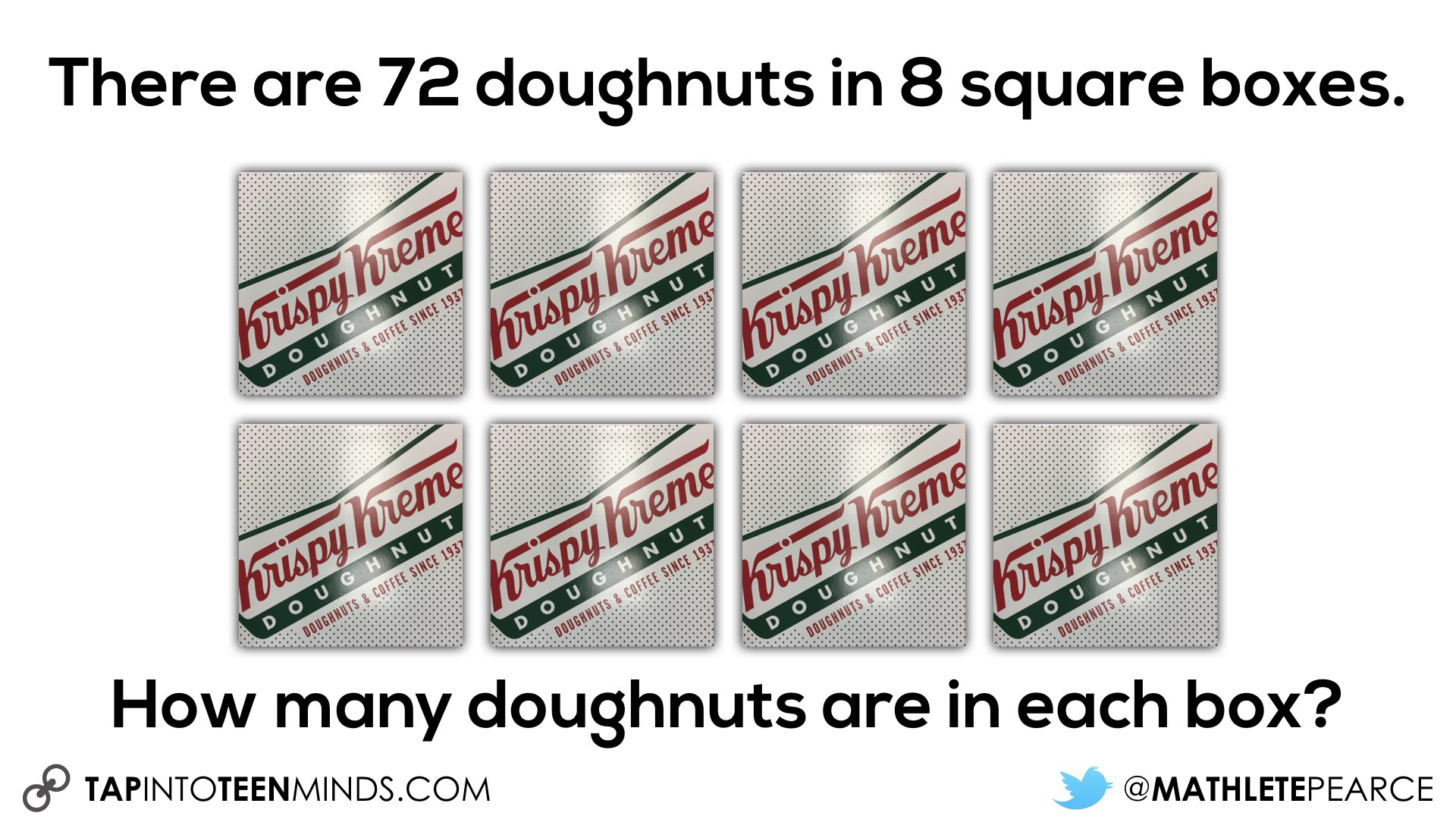In this case, students may use either ratio reasoning by scaling in tandem or rate reasoning by jumping straight to the number of doughnuts in each box by taking the quotient.

If a student uses ratio reasoning, scaling in tandem might look something like this using a concrete and/or visual representation: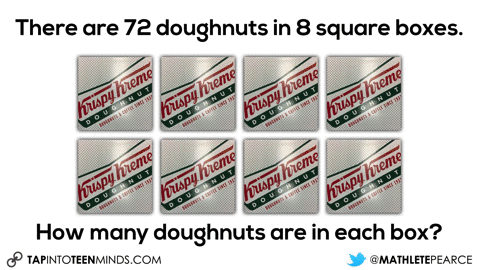Students using rate reasoning might look something like the following. I’ve also shown the visual and symbolic representations side-by-side: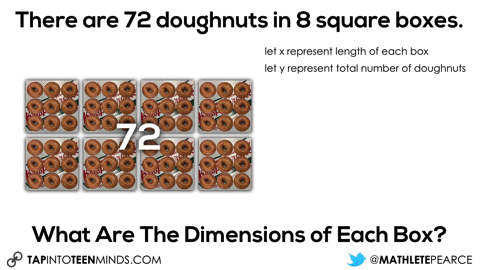Now that we’ve introduced square boxes, we might consider playing in the land of single-row boxes and square boxes for students to do some algebraic thinking and problem solving.Here, we can have students make a prediction noting that the images are to scale and thus they can use the size of the single doughnut to help them with that prediction.

Having them share with their partner how they came up with their prediction can be extremely useful to see what they notice about each of the boxes. The goal here would be for students to make the connection that each doughnut “strip” box is the same length as the square box dimensions.

You can then share some more information and have them update their prediction: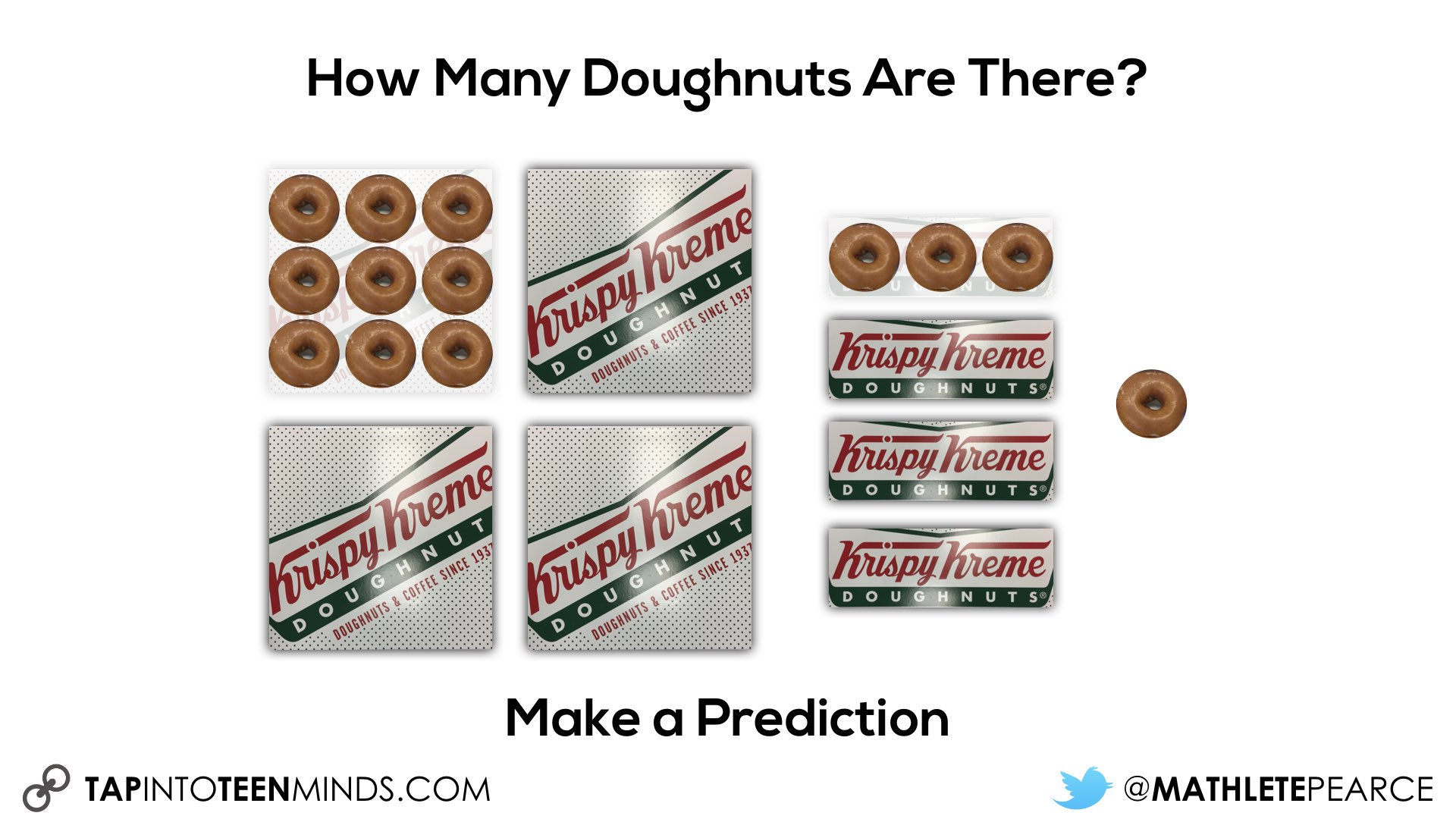Giving students a concrete representation of this situation by cutting out these shapes on card stock could be extremely useful for them to tangibly work with these quantities. If students are given the opportunity to manipulate these boxes of doughnuts, they may realize that they can create a complete rectangle (or square in this case) and that can also be helpful for their prediction.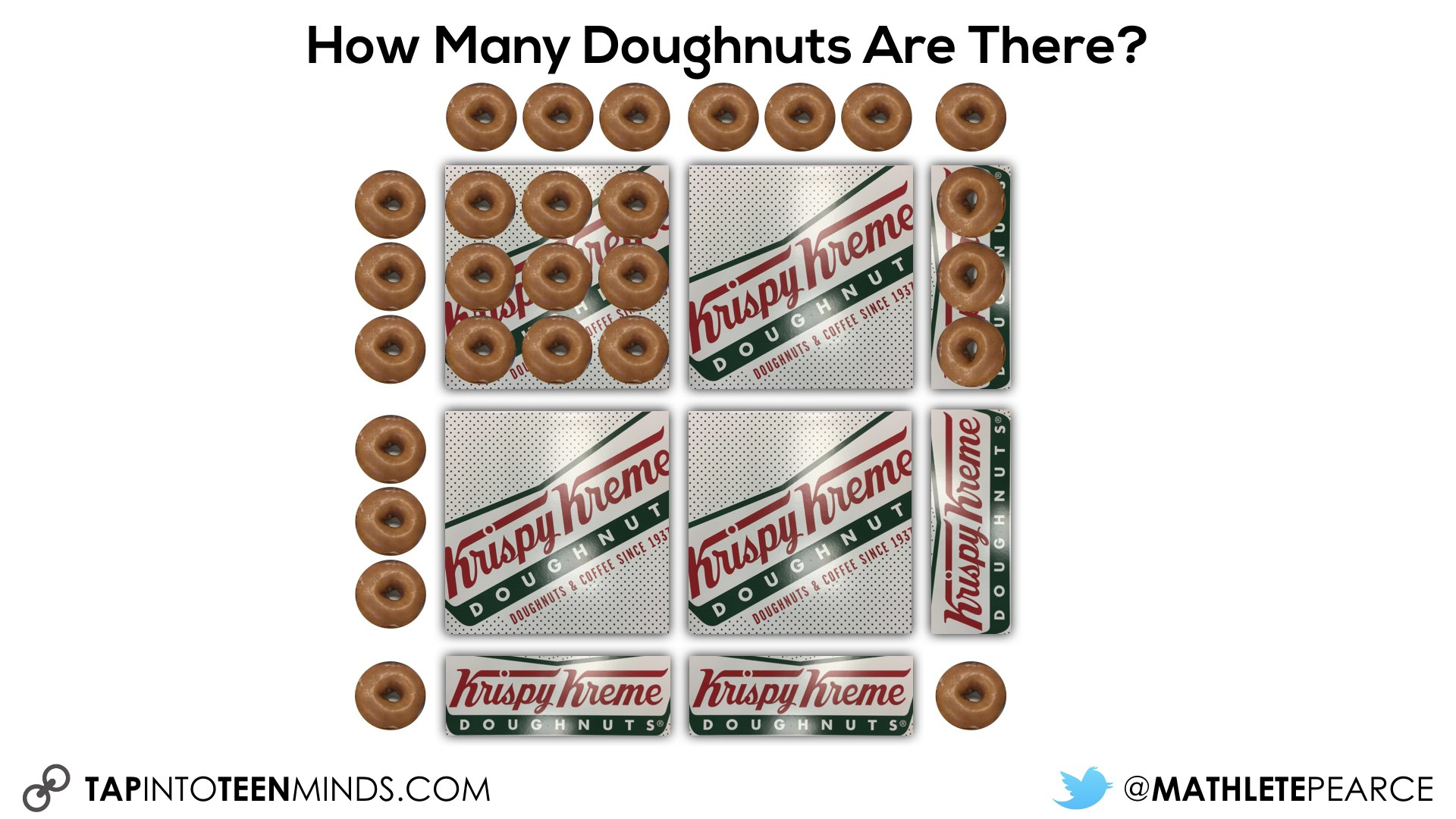By creating this rectangle, they can more easily come up with a total number of doughnuts using their knowledge of arrays and area models.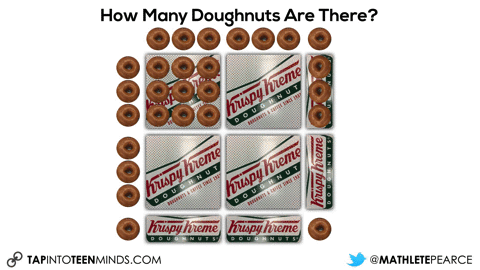Then, we can allow students to use their same manipulatives to determine the total number of doughnuts in this situation. Same size boxes, just more of them.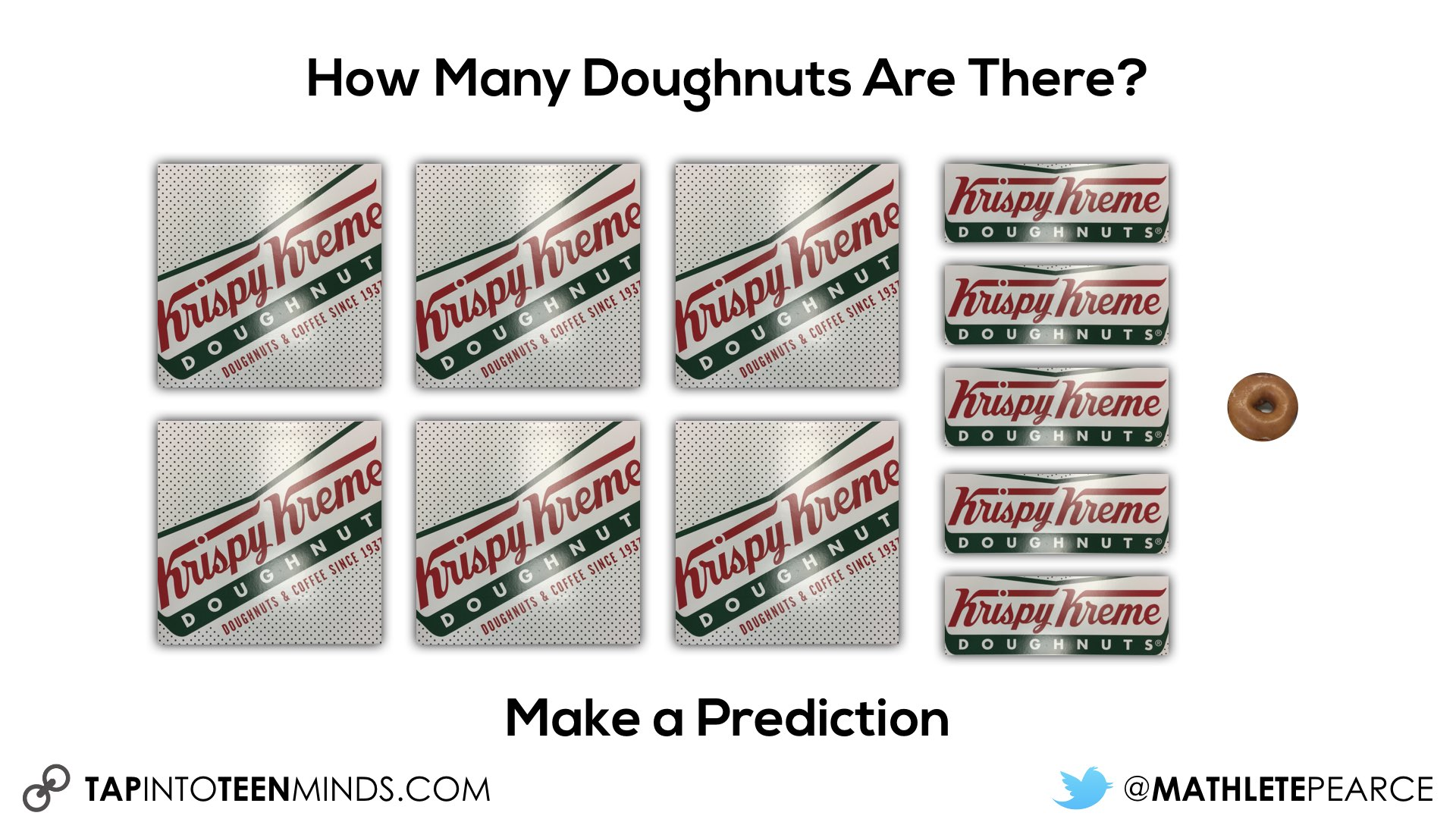Over time, we would want students to start noticing patterns and using those patterns to help them come to a total using multiplicative and algebraic thinking.

Initially, they may do this verbally by describing the 6 square boxes, the 5 single row “sleeves” of doughnuts and the 1 extra doughnut. Since they know that there are 9 doughnuts in each square box and 3 doughnuts in each single row “sleeve”, it might sound like this:

6 boxes of 9 doughnuts plus 5 boxes of 3 doughnuts plus 1 doughnut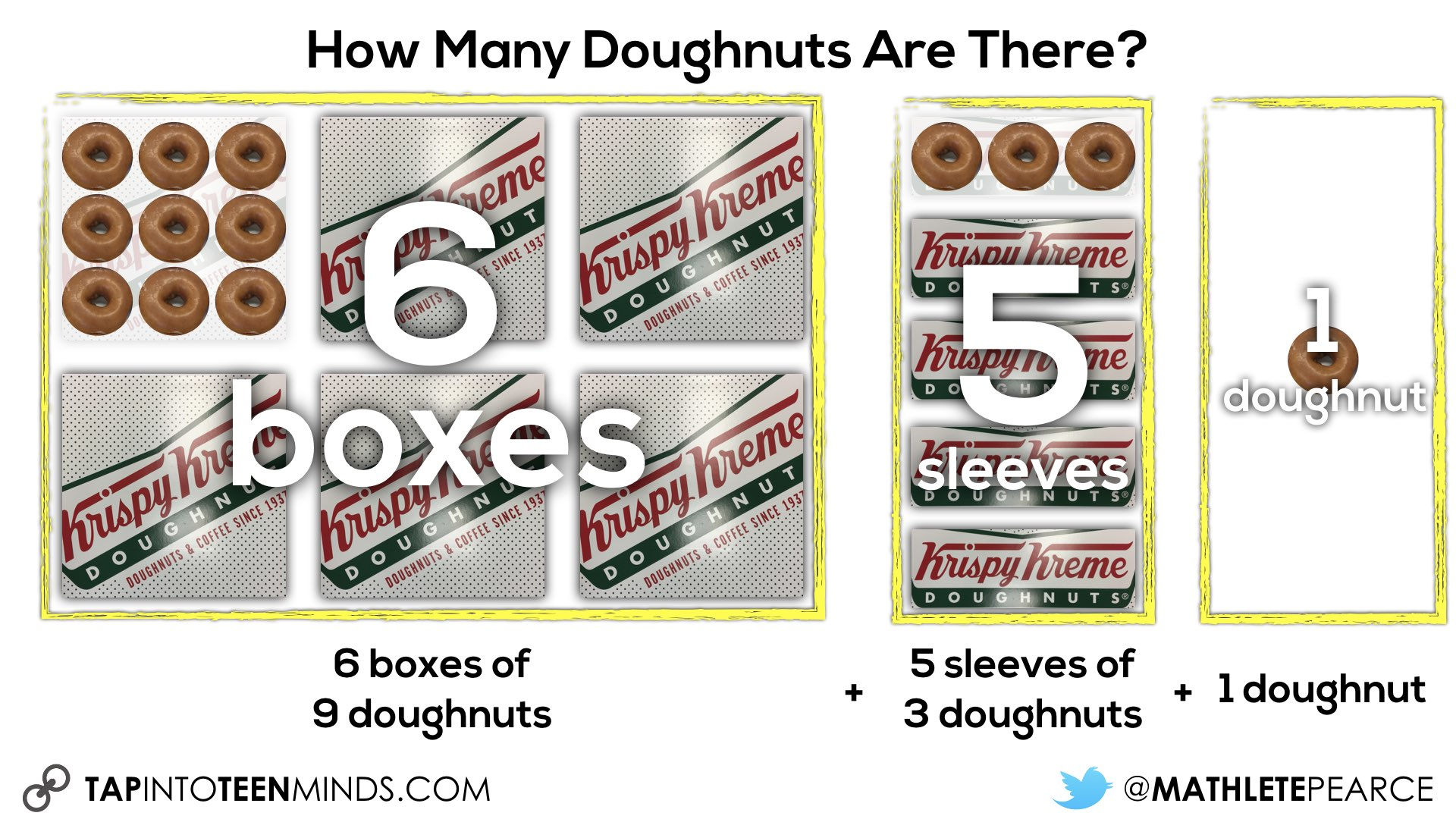We can help students to write their expression in words and then eventually, using symbols.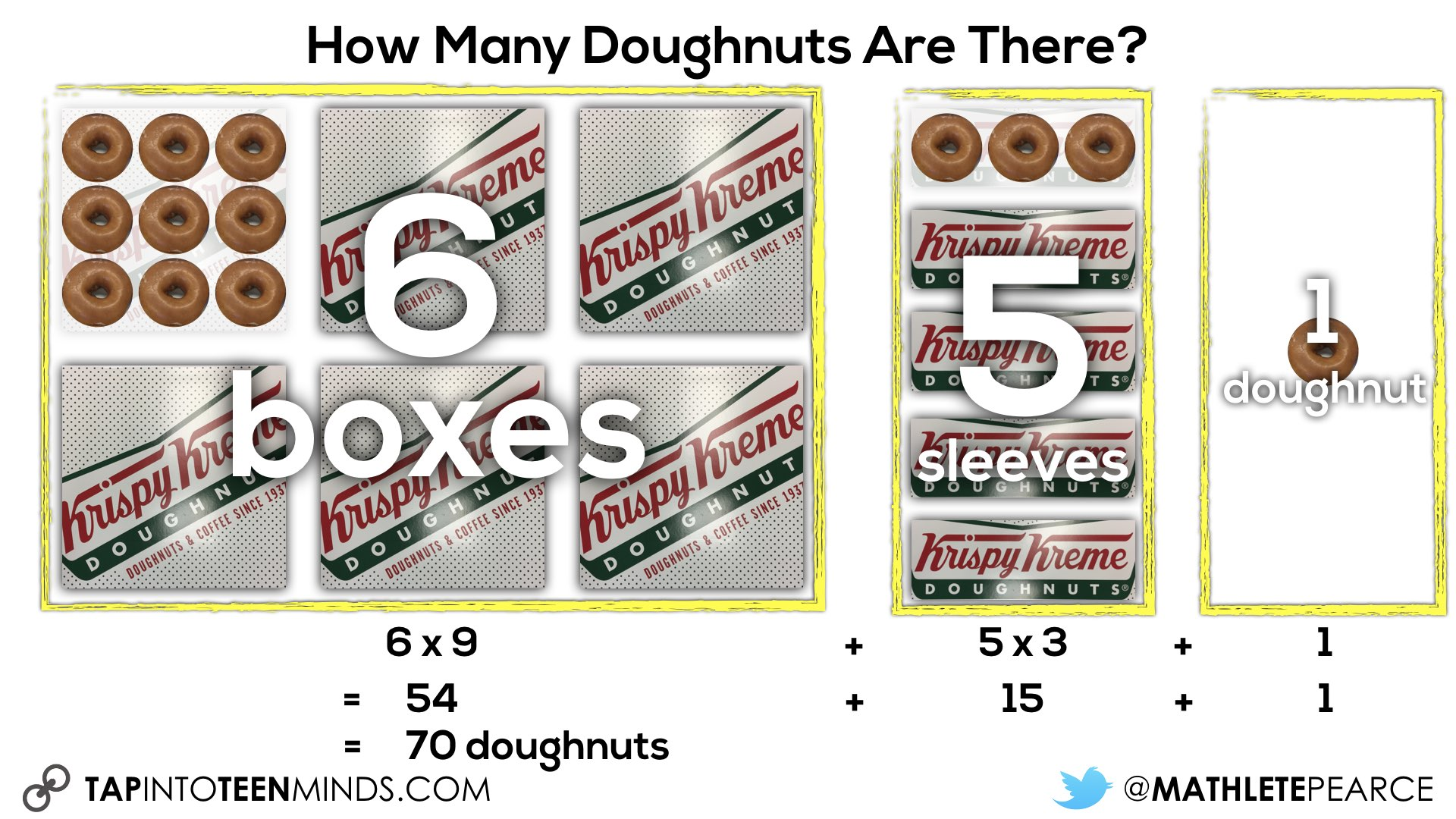After having quite a bit of experience doing this type of problem puzzle, we can have them start using algebraic equations using variables and in this case, even explicitly draw out how the squares are literally the single row “strips” of doughnuts “squared”.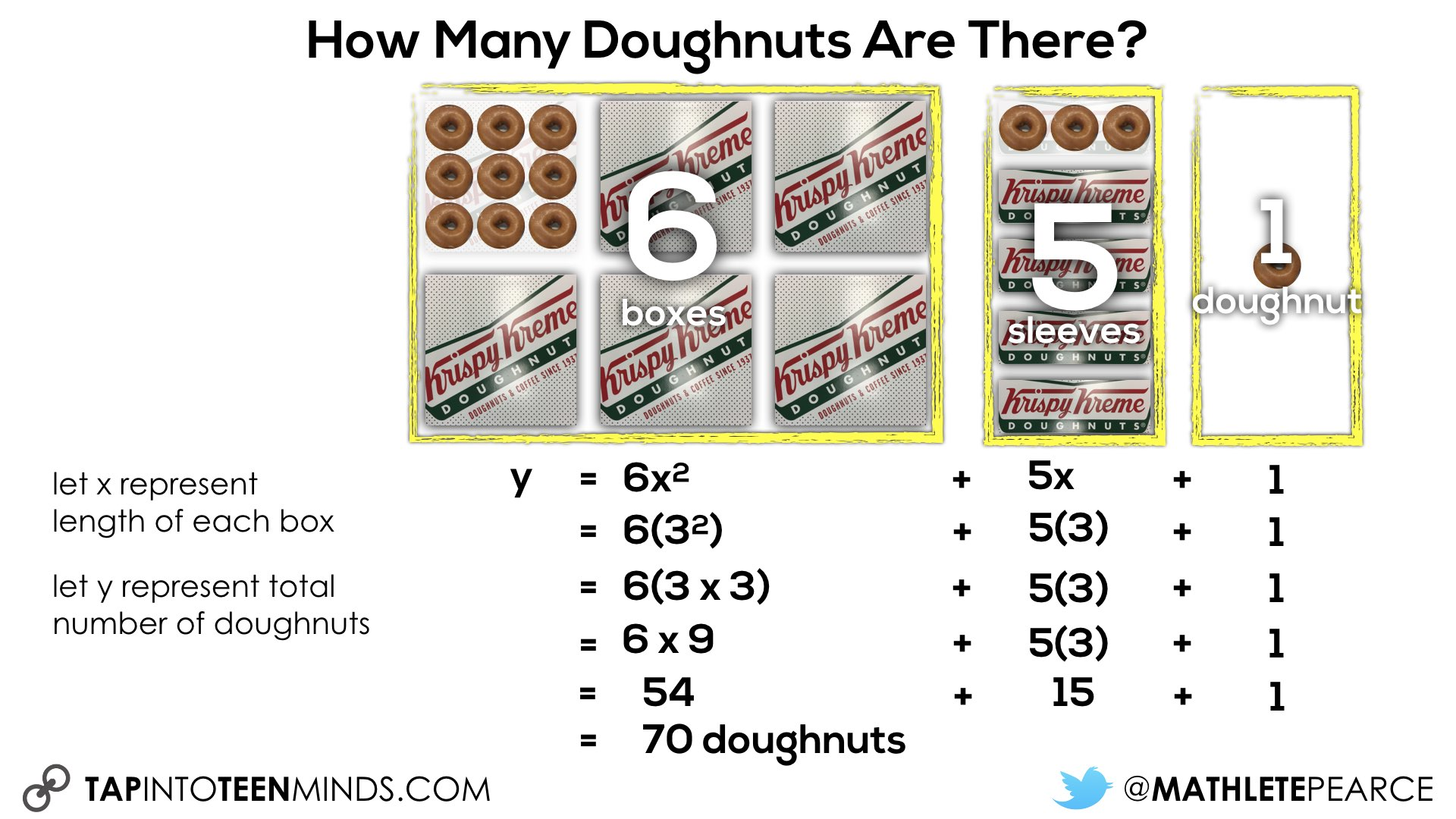Now that students have come up with an algebraic equation, we could then play with the dimensions of the boxes and have students utilize the equation to come up with solutions. For example, if we give students larger boxes, but keep the number of boxes the same, they can leverage the same equation.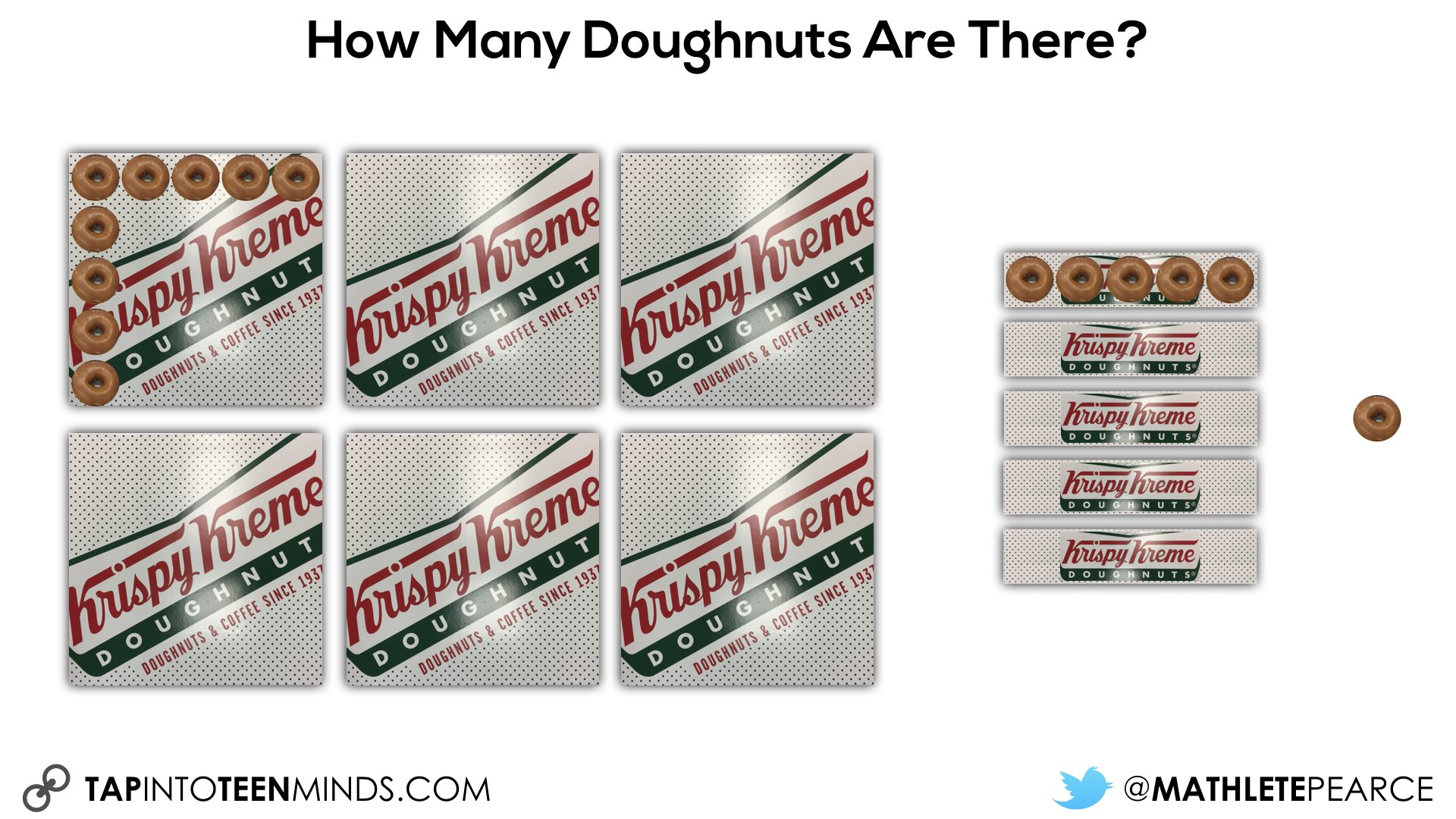Some students may still require time to play with this idea in words and then numerically, while others may feel comfortable jumping straight to the algebraic equation. However, in either case, the task is very accessible by starting concrete and leveraging visuals throughout regardless of whether they are working with symbols with more abstract representations.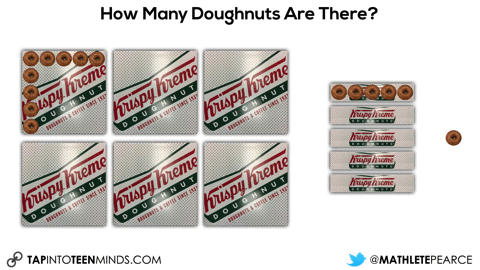When most students are showing signs of being ready to make the leap towards more abstract thinking, I’ll change the dimensions again to have students make a prediction.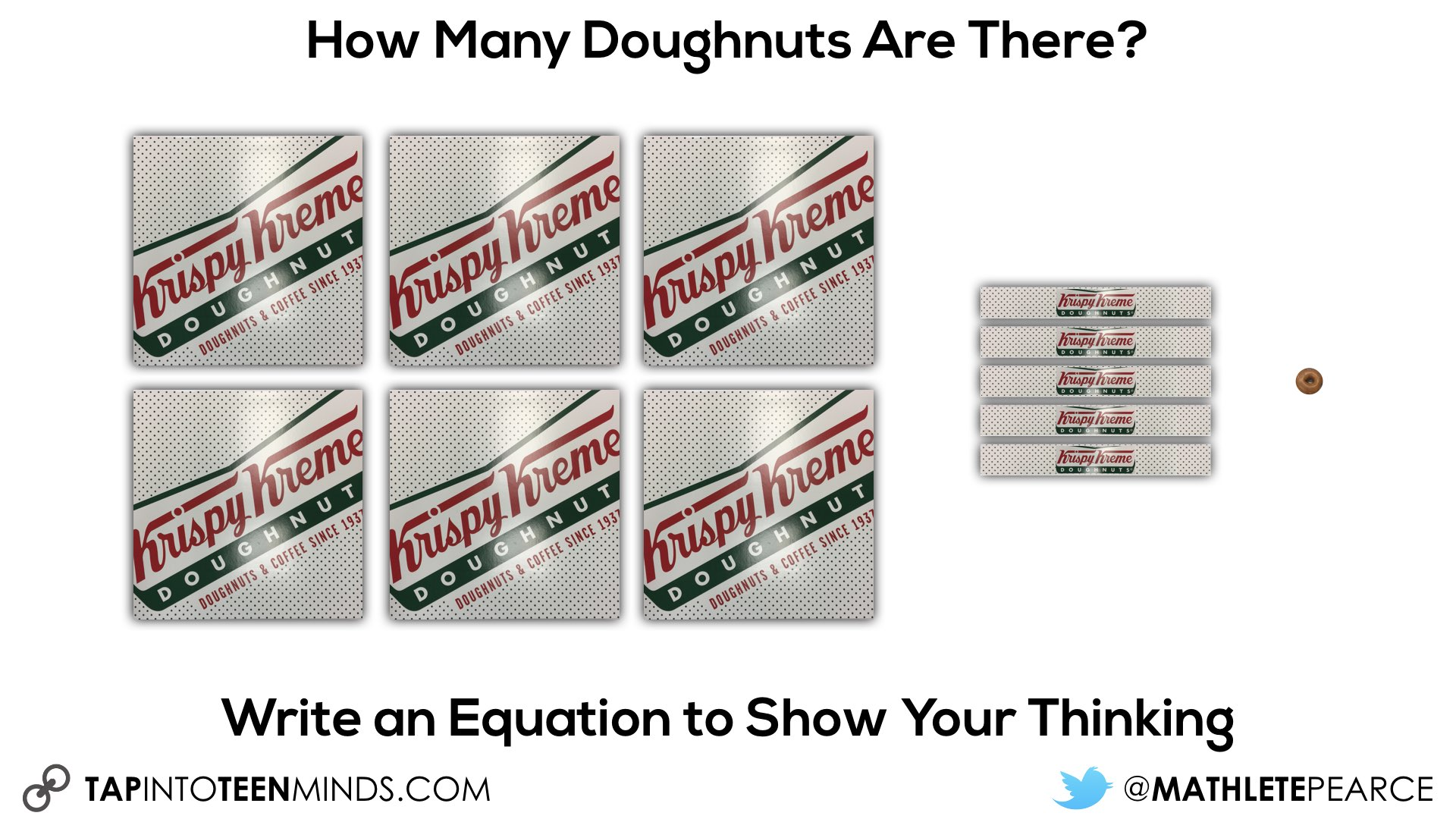We want to make sure that we help students explicitly make a connection between the doughnut “strip” boxes and the square boxes so they can see the connection to algebra tiles.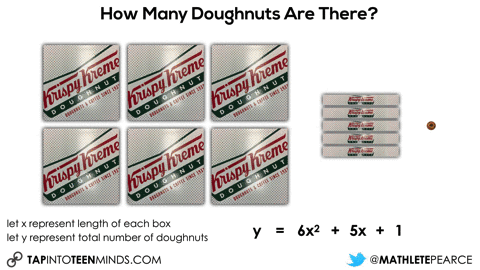In this particular example, not only can we play in the land of substituting values into algebraic equations and simplifying, but we can also connect to quadratic relationships and factoring trinomials.

Here’s a visual of substituting a value of x into a quadratic equation and simplifying: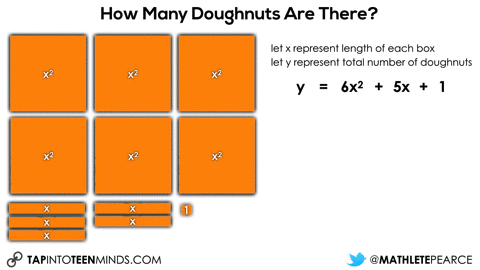By taking that same quadratic relationship and arranging as the familiar rectangle we are always trying to create when multiplying with base 10 blocks, we can easily see the two factors that are equivalent to this trinomial: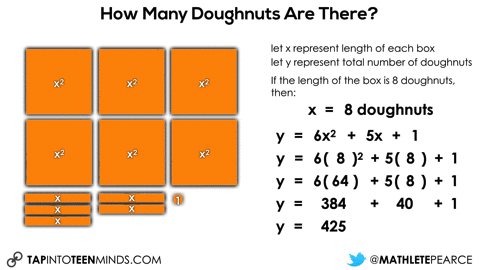Here’s what concreteness fading might look like in those grade 8 to grade 10 classrooms focusing on expanding and factoring polynomials: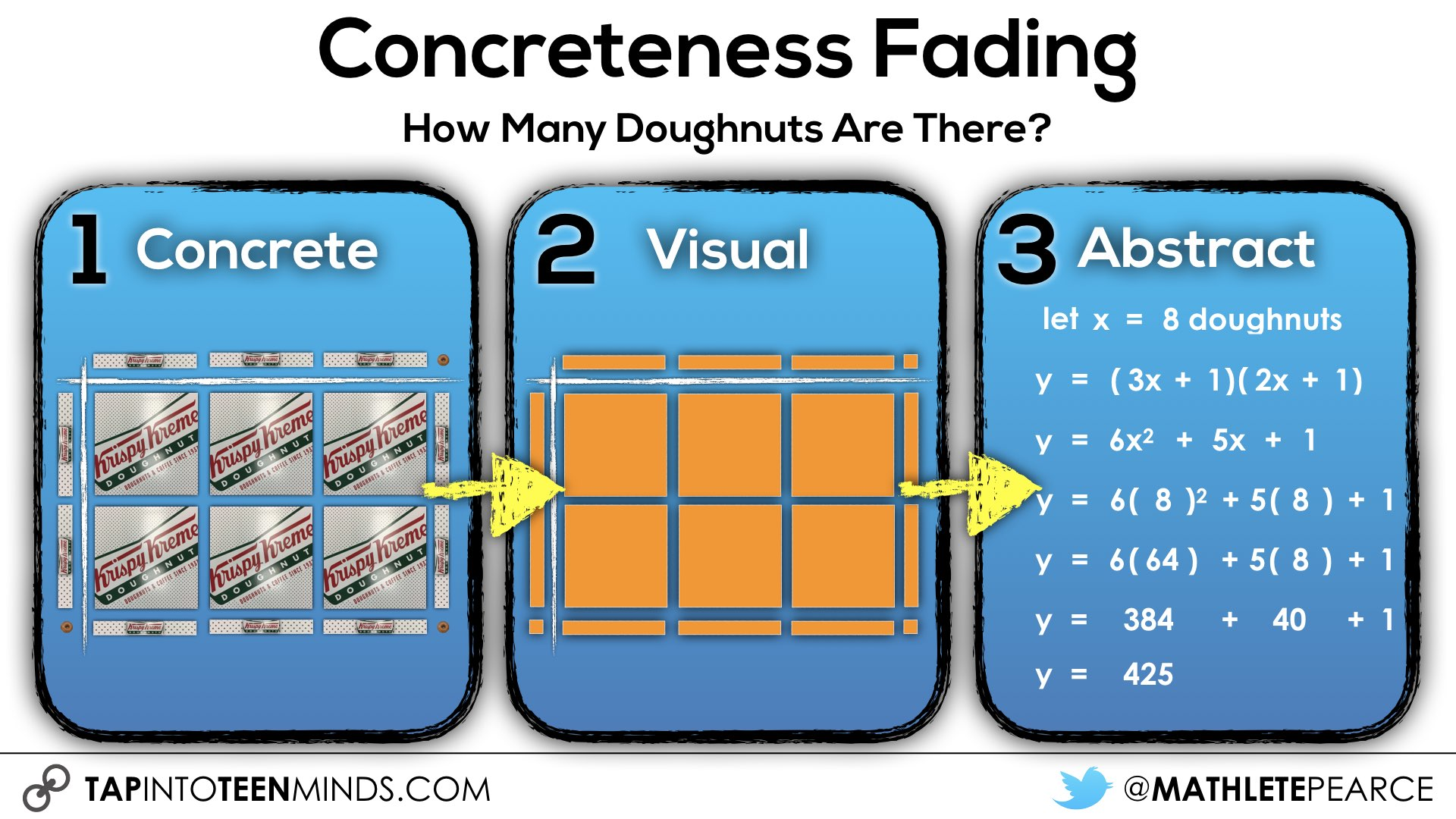Whew… We just started this post down in primary grades and worked our way up to high school math all through the use of concreteness fading. While the context in this last example might have been a tad contrived, I hope it helps us see how flexible mathematics really can be and how we might consider lowering the floor through the use of concrete and visual representations.

For years, I was spinning my wheels trying to teach students how to make sense of mathematics through abstract representations. However, even musicians are aware of the importance of marketing through a concreteness fading model.

Take the musician Prince for example.

Every successful musician knows that the best way to build a true fan base is to begin at the concrete phase. If you go to see Prince live in concert for example, you will quickly understand why he and his music are so great.

After seeing the show, you might rush to grab the next best thing to seeing him live in order to bring back the energy and positive feelings you had while watching live. Buying albums, videos, posters and magazines are great examples of how we can listen and see Prince in our minds as if he were there in the flesh.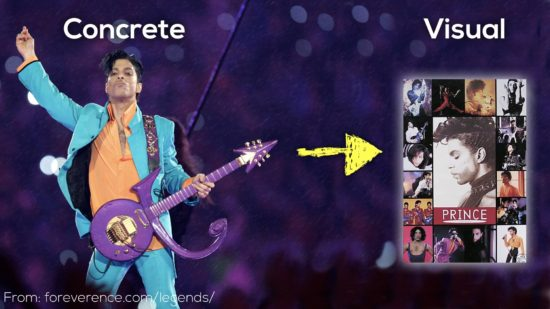For those who are fans of Prince, it is highly likely that you know that after years of being known as Prince, he would legally change his name to a symbol:This would be a career ending move if he didn’t already have millions of fans who had watched him live in concert (concrete) and enjoyed his music and memorabilia (visual). But yet, in math class, we so often begin with symbols and try to make meaning of them after.

My call to action here is to be more Prince and think about concreteness fading during the planning process of each and every lesson. If we do this, we stand a much better chance of Making Math Moments That Matter for students.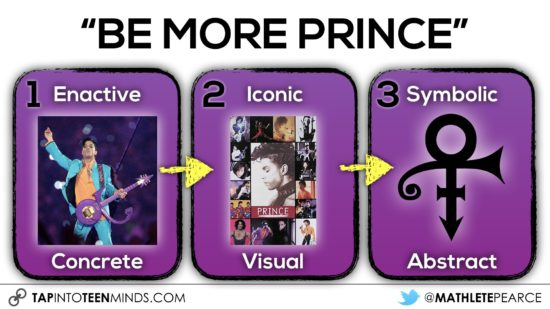How are you using concreteness fading in your lessons? I’d love to hear from you in the comments.

## Memorization vs. Automaticity

### What's The Difference?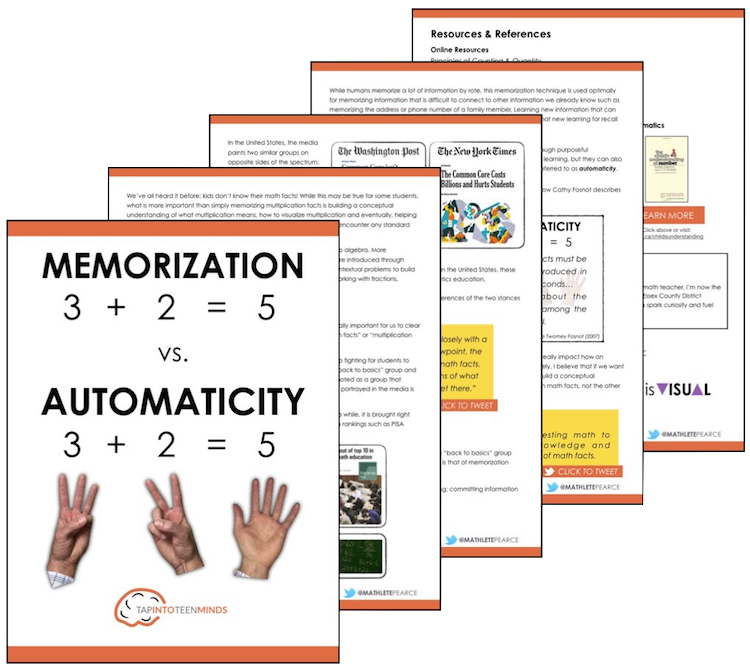## Share With Your Learning Community:I’m Kyle Pearce and I am a former high school math teacher. I’m now the K-12 Mathematics Consultant with the Greater Essex County District School Board, where I uncover creative ways to spark curiosity and fuel sense making in mathematics. Read more.

## Not What You're Looking For?

### Search By Criteria:

Spark Curiosity!